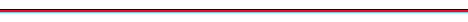# My Research Papers

All papers here are in pdf format (unless otherwise stated)

•  Two body problem on a sphere in the presence of a uniform magnetic field.
•  Attracting and repelling 2-body problems on a family of surfaces of constant curvature.
•  Generalized point vortex dynamics on CP2.
•  Non-Abelian momentum polytopes for products of CP2.
•  Integrability and dynamics of the n-dimensional symmetric Veselova top.
•  Persistence of stationary motion under explicit symmetry breaking perturbation.
•  Hermitian flag manifolds and orbits of the Euclidean group.
•  Reduction and relative equilibria for the 2 body problem on spaces of constant curvature.
•  Feynman path integrals and Lebesgue-Feynman measures.
•  Gauge momenta as Casimir functions of nonholonomic systems.
•  Periodic orbits in Hamiltonian systems with involutory symmetries.
•  Transformations of Feynman path integrals and the generalized densities of Feynman pseudomeasures.
•  Hamiltonian relative equilibria with continuous isotropy
(with Miguel Rodriguez-Olmos) [ArXiv]
•  Existence of symmetric central configurations.
•  Transformations of measures via their generalized densities.
•  Point vortices on the hyperbolic plane.
•  Bifurcations of relative equilibria near zero momentum in Hamiltonian systems with spherical symmetry.
•  Classification of symmetry groups for planar n-body choreographies.
•  A sufficient condition for the existence of Hamiltonian bifurcations with continuous isotropy.
•  Deformation of geometry and bifurcations of vortex rings
•  Finite-dimensional behaviour and observability in a randomly forced PDE
(with Dave Broomhead, Jerry Huke, and Mark Muldoon) [mims eprint, DOI]
•  Generalized Dirichlet to Neumann operator on invariant differential forms and equivariant cohomology.
•  Witten-Hodge theory for manifolds with boundary and equivariant cohomology.
•  Point Vortices on the Sphere: Stability of Symmetric Relative Equilibria
(with Frédéric Laurent-Polz and Mark Roberts) [mims eprint, DOI] (new version)
•  Dynamics of poles with position-dependent strengths and its optical analogues.
•  On the stability of Hamiltonian relative equilibria with non-trivial isotropy.
•  Symplectic group actions and covering spaces
•  A note on the geometry of linear Hamiltonian systems of signature 0 in R4
•  Stability of relative equilibria of point vortices on the sphere
(with Frédéric Laurent-Polz and Mark Roberts) (new version above, )
•  Bifurcation and forced symmetry breaking in Hamiltonian systems.
•  The relation between local and global dual pairs.
(with Juan-Pablo Ortega and Tudor Ratiu) [mims eprint]
•  Golden gaskets: variations on the Sierpinski sieve.
•  Relative periodic orbits of symmetric Lagrangian systems.
(with Chris McCord, Mark Roberts and Luca Sbano) [Abstract, Paper]
•  Vortex dynamics on cylinders.
(with Anik Soulière and Tadashi Tokieda) [Paper, DOI]
•  Openness of momentum maps and persistence of extremal relative equilibria.
•  Group theoretic conditions for existence of robust relative homoclinic trajectories.
•  Relative equilibria of point vortices on the sphere.
(with Chjan Lim and Mark Roberts) [eprint, DOI]
•  A note on semisymplectic actions of Lie groups.
•  Real continuation from the complex quadratic family: Fixed point bifurcation sets.
•  Perturbing a symmetric resonance: the magnetic spherical pendulum.
•  Relative equilibria of molecules.
•  Multiplicities of zero-schemes in quasi-homogeneous corank-1 singularities.
•  Persistance d'orbites périodiques relatives dans les systèmes hamiltoniens symétriques.
•  Persistence and stability of relative equilibria.
•  Bifurcation générique d'ondes rotatives d'isotropie maximale.
(with Pascal Chossat and Muriel Koenig) [Abstract, Paper (145K)]
•  The path formulation of bifurcation theory.
•  Deformations of maps on complete intersections, Damon's KV-equivalence and bifurcations
(with David Mond) [Paper (222K)]
•  Multiplicities of critical points of invariant functions
•  Quotient spaces and critical points of invariant functions for C*-actions
•  On generic composites of mappings.
•  Caustics in time reversible Hamiltonian systems.
•  One-forms on singular curves and the topology of real curve singularities
(with Duco van Straten) [DOI]
•  Stability of nonlinear normal modes in symmetric Hamiltonian systems
•  Existence of nonlinear normal modes in symmetric Hamiltonian systems
•  Periodic solutions near equilibria of symmetric Hamiltonian systems
•  Non-linear normal modes of symmetric Hamiltonian systems
(with Mark Roberts and Ian Stewart) [Abstract]
•  Surfaces in 3-space and their contact with circles.
•  On contact between submanifolds.
•James Montaldi